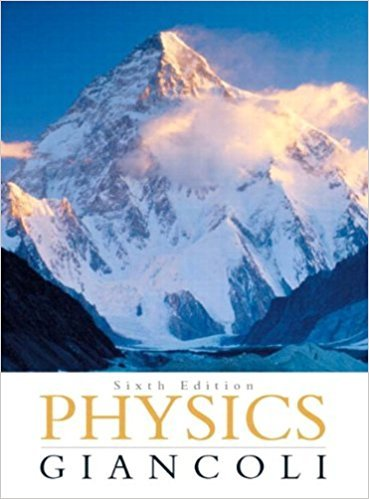×
×

# A particle revolves in a horizontal circle of radius 2.90ISBN: 9780321569837 99

## Solution for problem 27 Chapter 5

Physics: Principles with Applications | 6th Edition

• Textbook Solutions
• 2901 Step-by-step solutions solved by professors and subject experts
• Get 24/7 help from StudySoup virtual teaching assistantsPhysics: Principles with Applications | 6th Edition

4 5 1 285 Reviews
18
5
Problem 27

A particle revolves in a horizontal circle of radius 2.90 m. At a particular instant, its acceleration is 05 m/s2, in a direction that makes an angle of 32.0 to its direction of motion. Determine its speed (a) at this moment, and (/>) 2.00 s later, assuming constant tangential acceleration.

Step-by-Step Solution:
Step 1 of 3

Kinematics Position, Distance, and Displacement ● Before describing motion ○ Set up a coordinate system, define an origin and positive direction, define position ● Position: where ● Distance: total of how far you go ● Displacement:...

Step 2 of 3

Step 3 of 3

##### ISBN: 9780321569837

The answer to “A particle revolves in a horizontal circle of radius 2.90 m. At a particular instant, its acceleration is 05 m/s2, in a direction that makes an angle of 32.0 to its direction of motion. Determine its speed (a) at this moment, and (/>) 2.00 s later, assuming constant tangential acceleration.” is broken down into a number of easy to follow steps, and 50 words. This full solution covers the following key subjects: its, acceleration, direction, later, constant. This expansive textbook survival guide covers 33 chapters, and 4141 solutions. Since the solution to 27 from 5 chapter was answered, more than 342 students have viewed the full step-by-step answer. This textbook survival guide was created for the textbook: Physics: Principles with Applications, edition: 6. The full step-by-step solution to problem: 27 from chapter: 5 was answered by , our top Physics solution expert on 09/09/17, 04:43AM. Physics: Principles with Applications was written by and is associated to the ISBN: 9780321569837.

Unlock Textbook Solution